Courses

# Ideal and Lost Work for Flow Systems Civil Engineering (CE) Notes | EduRev

## Civil Engineering (CE) : Ideal and Lost Work for Flow Systems Civil Engineering (CE) Notes | EduRev

The document Ideal and Lost Work for Flow Systems Civil Engineering (CE) Notes | EduRev is a part of the Civil Engineering (CE) Course Thermodynamics.
All you need of Civil Engineering (CE) at this link: Civil Engineering (CE)

Ideal and Lost Work for Flow Systems

We next derive the expressions for work exchange between system and surroundings for an open system operating under steady state by incorporating the idea of irreversibility. As we have discussed earlier, mechanical irreversibilities lead to loss of work due to dissipative conversion to heat. Thus, if work is to be delivered by an open system the maximum work obtains when the processes are mechanically reversible, we call that ideal work. Conversely, when work is done on the system the ideal work provides the minimum work needed to change the fluid state between the inlet and the exit. This is because an extra work would need to be provided beyond the ideal work against mechanical dissipative forces. From the considerations in the last section it may be evident that ideal work obtains when the processes associated with the open system are both internally and externally reversible. For such a case one may write eqn. 4.29 as follows: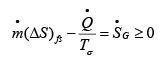..(4.30)

Thus: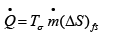..(4.31)

From the 1st Law applied to the ideal case: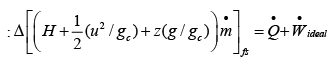..(4.32)

Putting eqn. 4.31 into 4.32 and simplifying (assuming negligible kinetic and potential energy changes):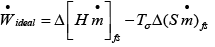..(4.33)

Or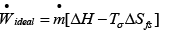..(4.34)

In real processes however, actual work involved will depend on the extent of associated irreversibilities; hence we define lost work as follows: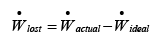..(4.35)

Now from eqn. 4.32 for the simplest case where kinetic and potential energy changes are negligible: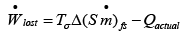..(4.36)

Using eqns. 4.34 and 4.35: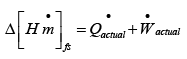..(4.37)

Applying eqn. 4.30, to a real process: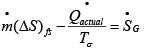..(4.38)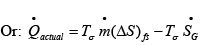..(4.39)

Thus from eqns. 4.37 and 4.39 it follows that: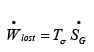..(4.40)

Eqn. 4.40 suggests that greater the entropy generation rate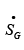due to process irreversibility, greater is the lost work. Since irreversibilities implicit in a process cannot be calculated theoretically, it is indirectly expressed by a process efficiency factor, η . The expression for such efficiency is as follows.

For work done by system,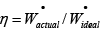..(4.41)

For work done on a system,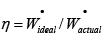..(4.42)

Entropy: A Statistical Thermodynamic Interpretation

As with internal energy the concept of entropy may be provided a microscopic, molecular interpretation. However, it may be noted that unlike internal energy, molecules do not possess entropy. Entropy is purely a measure thermodynamic probability associated with the distribution of various positional and energy states that molecules of a substance may exist under given conditions of temperature and pressure. To understand this, consider a simple system comprised of a rigid, insulated vessel divided into two compartments of equal volume by a partition (of negligible volume, fig. A.4.1). Initially, the left compartment contains gas at a temperature T1 and pressure P1 , and the volume of each compartment is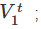the right compartment is fully evacuated. The system is at equilibrium under the above conditions, and is described by the ideal.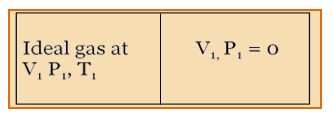gas EOS. At a certain point of time the partition is ruptured and the gas contained in the left compartment allowed to fill the entire system volume. Eventually the gas would reach a new equilibrium state (2) that is characterized by a pressure P1 / 2 throughout. For the above process, applying the first law:
∆U = Q+W
But: Q = W = 0
Hence, ∆U = 0       ...(A.4.1.1)

This implies that for the gas initially on the left side: ∆T= 0; or T2 =T1

The entropy change per mole of the gas then is given by eqn. 4.21:

Thus,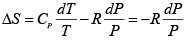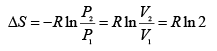(A.4.1.2)

From a microscopic point of view the molecular state variables are: position and energy. Since there is no change of temperature during the expansion process, the internal energy of each molecule does not change. There, is, however, a possible change in the position occupied a molecule. After the expansion a molecule may be located either on the left half or in the right half of the compartment at any point of time. We may say, in a simplified sense, there are two possible positions that it may have. Considering one mole of gas as basis, if there are, in general, j such states (either of position or energy) that a molecule may exist in, the total number of ways that an Avogadro number of molecules ( N =  6.023 x 1023 ) may be distributed over all the available states is given by (with n j molecules in the jth state);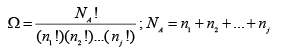(A.4.1.3)

The notation used for the right side of the above equation, Ω is called the thermodynamic probability. Thus for the system in question, in the initial state all the molecules are located in the left half of the compartment, so there is only ‘one’ positional state; thus: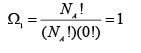(A.4.1.4)

However, in the final state as there are two possible positional states, the corresponding maximum thermodynamic probability is:(A.4.1.5)

The description ‘maximum’ is important, as the exact number of molecules that may be distributed between the two compartments at any point of time need not be as the same, as assumed in eqn. A.4.1.5. In general there could be many possible bifurcations of the total number molecules into the two compartments, each characterized by a corresponding thermodynamic probability. However, simple calculations suggest that Ω2 has the highest possible value if each compartment contains equal number of molecules, i.e., NA/2. Indeed, intuitively speaking, one would expect that to be the most likely distribution in the second equilibrium state. This implies that the system would tend to move to an equilibrium state that has the highest of all the possible values of the thermodynamic probability that one may attribute to the system. It was Ludwig Eduard Boltzmann (1844–1906) the Austrian physicist, who is credited with the development of the statistical concept of nature (now known as statistical mechanics), first proposed the relation between entropy and thermodynamic probability:

S = k ln Ω                  ..... (A.4.1.6)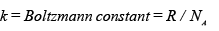If one applies the equation to the expansion process, one obtains the following relation: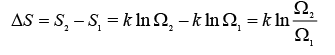..... (A.4.1.7)

Thus: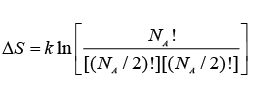or: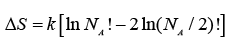..... (A.4.1.8)

By the well-known Stirling’s formula, or large values of an integer N, one has:

ln N ! = N ln N −N                    ..... (A.4.1.9)

On applying eqn. A. in A.4.1.8 and simplifying the following result obtains: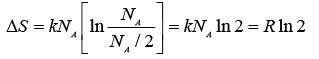..... (A.4.1.10)

Thus we see that the results of both eqns. A.4.1.2 and A.4.1.10 are identical. There is thus a convergence between the macroscopic and microscopic description of the concept of entropy.

It is particularly significant that the above result obtains if one assumes the highest value of Ω2 as its most likely value. It may be argued that the highest value of Ω2 corresponds to the most disordered state at the molecular level. Indeed extending the argument, since Ω1= 1 the initial state is more ordered than state 2, as in the former all molecules are characterized by a single position coordinate, and therefore the probability of finding a molecule in that position is unity; in other words one has complete (or ‘certain’) information about position of any molecule. In contrast in the second state the probability of finding a molecule at any one position is half. This obtains if each compartment contains exactly half the total number of molecules. Any other distribution would be less disordered than this state, and also less probable! This is the reasoning which lies at the root of the popular description of entropy as a measure of disorder at the molecular level. It follows that a system at non-equilibrium state (say, at the point of rupture of the partition) always tends to the most disordered molecular state when it attains a new equilibrium. Since one has less microscopic information about a system with a greater degree of disorder, ‘information’ is also described as negentropy.

Offer running on EduRev: Apply code STAYHOME200 to get INR 200 off on our premium plan EduRev Infinity!

## Thermodynamics

56 videos|92 docs|33 tests

,

,

,

,

,

,

,

,

,

,

,

,

,

,

,

,

,

,

,

,

,

;